# relativity

Also found in: Dictionary, Thesaurus, Wikipedia.
Related to relativity: special relativity, Relativity physics

## relativity,

physical theory, introduced by Albert Einstein, that discards the concept of absolute motion and instead treats only relative motion between two systems or frames of reference. One consequence of the theory is that space and time are no longer viewed as separate, independent entities but rather are seen to form a four-dimensional continuum called space-timespace-time,
central concept in the theory of relativity that replaces the earlier concepts of space and time as separate absolute entities. In relativity one cannot uniquely distinguish space and time as elements in descriptions of events.
. Full comprehension of the mathematical formulation of the theory can be attained only through a study of certain branches of mathematics, e.g., tensor calculus. Both the special and general theories have been established and accepted into the structure of physics. Einstein also sought unsuccessfully for many years to incorporate the theory into a unified field theory valid also for subatomic and electromagnetic phenomena.

### Evolution from Classical Theory

The modern theory is an extension of the simpler Galilean or Newtonian concept of relativity, which holds that the laws of mechanics are the same in one system as in another system in uniform motion relative to it. Thus, it is impossible to detect the motion of a system by measurements made within the system, and such motion can be observed only in relation to other systems in uniform motion. The older concept of relativity assumes that space and time are correctly measured separately and regards them as absolute and independent realities. The system of relativity and mechanics of Galileo and Newton is perfectly self-consistent, but the addition of Maxwell's theory of electricity and magnetism to the system leads to fundamental theoretical difficulties related to the problem of absolute motion.

It seemed for a time that the etherether
or aether,
in physics and astronomy, a hypothetical medium for transmitting light and heat (radiation), filling all unoccupied space; it is also called luminiferous ether. In Newtonian physics all waves are propagated through a medium, e.g.
, an elastic medium thought to be present throughout space, would provide a method for the measurement of absolute motion, but certain experiments in the late 19th cent. gave results unexplained by or contradicting Newtonian physics. Notable among these were the attempts of A. A. Michelson and E. W. Morley (1887) to measure the velocity of the earth through the supposed ether as one might measure the speed of a ship through the sea. The null result of this measurement caused great confusion among physicists, who made various unsuccessful attempts to explain the result within the context of classical theory.

### The Special Theory of Relativity

The validity of the classical concepts of absolute and independent time and space was challenged by H. A. Lorentz and others. Since absolute motion cannot be confirmed by objective measurement, Einstein suggested that it be discarded from physical reasoning; he explained the results of the Michelson-Morley experiment by means of the special relativity theory, which he enunciated in 1905. This theory accepts the hypothesis that the laws of nature are the same in different moving systems applies also to the propagation of light, so that the measured speed of light is constant for all observers regardless of the motion of the observer or of the source of the light. Einstein deduced from these hypotheses the full logical consequences and reformulated the mathematical equations of physics, basing them in part on equations of H. A. Lorentz (see Lorentz contractionLorentz contraction
, in physics, contraction or foreshortening of a moving body in the direction of its motion, proposed by H. A. Lorentz on theoretical grounds and based on an earlier suggestion by G. F.
) by which measurements made in one uniformly moving system can be correlated with measurements in another system if the velocity of one relative to the other is known.

The theory resolves the conflict between Newton's mechanics and Maxwell's electrodynamics by introducing fundamental changes in Newton's theory. In most phenomena of ordinary experience the results obtained from the application of the special theory approximate those based on Newtonian dynamics, but the results deviate greatly for phenomena occurring at velocities approaching the speed of light. In innumerable cases where the results predicted by these theories are incompatible, experimental evidence supports the Einstein theory. Among its assertions and consequences are the propositions that the maximum velocity attainable in the universe is that of light; that mass and energy are equivalent and interchangeable properties (this is spectacularly confirmed by nuclear fission, on which the atomic bomb is based); that objects appear to contract in the direction of motion; that the rate of a moving clock seems to decrease as its velocity increases; that events that appear simultaneous to an observer in one system may not appear simultaneous to an observer in another system; and that, since absolute time is excluded from physical reasoning because it cannot be measured, the results of observers in different systems are equally correct.

### The General Theory of Relativity

Einstein expanded the special theory of relativity into a general theory (completed c.1916) that applies to systems in nonuniform (accelerated) motion as well as to systems in uniform motion. The general theory is principally concerned with the large-scale effects of gravitationgravitation,
the attractive force existing between any two particles of matter. The Law of Universal Gravitation

Since the gravitational force is experienced by all matter in the universe, from the largest galaxies down to the smallest particles, it is often called
and therefore is an essential ingredient in theories of the universe as a whole, or cosmologycosmology,
area of science that aims at a comprehensive theory of the structure and evolution of the entire physical universe. Modern Cosmological Theories
. The theory recognizes the equivalence of gravitational and inertial massmass,
in physics, the quantity of matter in a body regardless of its volume or of any forces acting on it. The term should not be confused with weight, which is the measure of the force of gravity (see gravitation) acting on a body.
. It asserts that material bodies produce curvatures in space-time that form a gravitational field and that the path of a body in the field is determined by this curvature. The geometry of a given region of space and the motion in the field can be predicted from the equations of the general theory.

Details of the motions of the planet Mercury had long puzzled astronomers; Einstein's computations explained them. He stated that the path of a ray of light is deflected by a gravitational field; observations of starlight passing near the sun, first made by A. S. Eddington during an eclipse of the sun in 1919, confirmed this. He predicted that in a gravitational field spectral lines of substances would be shifted toward the red end of the spectrum. This has been confirmed by observation of light from white dwarfwhite dwarf,
in astronomy, a type of star that is abnormally faint for its white-hot temperature (see mass-luminosity relation). Typically, a white dwarf star has the mass of the sun and the radius of the earth but does not emit enough light or other radiation to be easily
stars. Further confirmation has been obtained in more recent years from precision measurements using artificial satellites, the Viking lander on Mars, and Gravity Probe B (designed specifically to test the theory) as well as from detailed observations of pulsars.

### Bibliography

See A. Einstein, The Meaning of Relativity (6th ed. 1956) and, with others, The Principle of Relativity (1923, repr. 1958; a collection of original papers on the theory); M. Gardner, Relativity for the Million (1962); D. Bohm, The Special Theory of Relativity (1965); J. Schwinger, Einstein's Legacy (1986).

## Relativity

A general theory of physics, primarily conceived by Albert Einstein, which involves a profound analysis of time and space, leading to a generalization of physical laws, with far-reaching implications in important branches of physics and in cosmology. Historically, the theory developed in two stages. Einstein's initial formulation in 1905 (now known as the special, or restricted, theory of relativity) does not treat gravitation; and one of the two principles on which it is based, the principle of relativity (the other being the principle of the constancy of the speed of light), stipulates the form invariance of physical laws only for inertial reference systems. Both restrictions were removed by Einstein in his general theory of relativity developed in 1915, which exploits a deep-seated equivalence between inertial and gravitational effects, and leads to a successful “relativistic” generalization of Isaac Newton's theory of gravitation.

#### Special theory

The key feature of the theory of special relativity is the elimination of an absolute notion of simultaneity in favor of the notion that all observers always measure light to have the same velocity, in vacuum, c, independently of their own motion. The impetus for the development of the theory arose from the theory of electricity and magnetism developed by J. C. Maxwell. This theory accounted for all observed phenomena involving electric and magnetic fields and also predicted that disturbances in these fields would propagate as waves with a definite speed, c, in vacuum. These electromagnetic waves predicted in Maxwell's theory successfully accounted for the existence of light and other forms of electromagnetic radiation. However, the presence of a definite speed, c, posed a difficulty, since if one inertial observer measures light to have velocity c, it would be expected that another inertial observer, moving toward the light ray with velocity v with respect to the first, would measure the light to have velocity c + v. Hence, it initially was taken for granted that there must be a preferred rest frame (often referred to as the ether) in which Maxwell's equations would be valid, and only in that frame would light be seen to travel with velocity c. However, this viewpoint was greatly shaken by the 1887 experiment of A. A. Michelson and E. W. Morley, which failed to detect any motion of the Earth through the ether. By radically altering some previously held beliefs concerning the structure of space and time, the theory of special relativity allows Maxwell's equations to hold, and light to propagate with velocity c, in all frames of reference, thereby making Maxwell's theory consistent with the null result of Michelson and Morley. See Electromagnetic radiation, Light, Maxwell's equations

#### Simultaneity in prerelativity physics

The most dramatic aspect of the theory of special relativity is its overthrowing of the notion that there is a well-defined, observer-independent meaning to the notion of simultaneity. The following terminology will be introduced: An event is a point of space at an instant of time. Since it takes four numbers to specify an event—one for the time at which the event occurred and three for its spatial position—it follows that the set of all events constitutes a four-dimensional continuum, which is referred to as space-time.

A space-time diagram (Fig. 1) is a plot of events in space-time, with time, t, represented by the vertical axis and two spatial directions (x, y) represented by the horizontal axes. (The z direction is not shown.) For any event A shown in the diagram, there are many other events in this diagram—say, an event B—having the property that an observer or material body starting at event B can, in principle, be present at event A. The collection of all such events constitutes the past of event A. Similarly, there are many events—say, an event C—having the property that an observer or material body starting at event A can, in principle, be present at event C. These events constitute the future of A. Finally, there remain some events in space-time which lie neither to past nor future of A. In prerelativity physics, these events are assumed to make a three-dimensional set, and they are referred to as the events which are simultaneous with event A.

In both prerelativity physics and special relativity, an inertial observer is one who is not acted upon by any external forces. In both theories it is assumed that any inertial observer, can build a rigid cartesian grid of meter sticks, all of which intersect each other at right angles. Observer may then label the points on this cartesian grid by the coordinates (x, y, z) representing the distance of the point from along the three orthogonal directions of the grid. A clock may then be placed at each grid point. In prerelativity physics, these clocks may be synchronized by requiring that they start simultaneously with each other. Any event in space-time may then be labeled by the four numbers t, x, y, z, where t is the time of the event as determined by the synchronized clock situated at that grid point. See Frame of reference

It is of interest to compare the coordinate labelings given to events in space-time by two inertial observers, and , who are in relative motion. The relationship occurring in prerelativity physics is called a galilean transformation. In the simple case where moves with velocity v in the x direction with respect to, and these observers meet at the event A labeled by (t, x, y, z) = (t, x, y, z) = (0, 0, 0, 0), with the axes of the grid of meter sticks carried by aligned (that is, not rotated) with respect to those of, the transformation is given by Eqs. (1).

(1{\it a})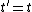(1{\it b})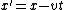(1{\it c})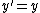(1{\it d})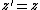The galilean transformation displays in an explicit manner that the two inertial observers, and , agree upon the time labeling of events and, in particular, agree upon which events are simultaneous with a given event.

#### Causal structure in special relativity

In special relativity there is a different causal relationship between an arbitrary event A and other events in space-time (Fig. 2). As in prerelativity physics, there are many events, B, which lie to the past of A. There also are many events which lie to the future of A. However, there is now a much larger class of events which lie neither to the past nor to the future of A. These events are referred to as being spacelike-related to A.

The most striking feature of this causal structure (Fig. 2) is the absence of any three-dimensional surface of simultaneity. Indeed, the closest analog to the surface of simultaneity in prerelativity physics is the double-cone-shaped surface that marks the boundaries of the past and future of event A. This surface comprises the paths in space-time of all light rays which pass through event A, and for this reason it is referred to as the light cone of A. Thus, the statement that the events lying to the future of A are contained within the light cone of A is equivalent to the statement that a material body present at event A can never overtake a light ray emitted at event A. In special relativity, the light cone of an event A replaces the surface of simultaneity with event A as the absolute, observer-independent structure of space-time related to causality.

As in prerelativity physics, it is assumed in special relativity that an inertial observer, can build a rigid grid of meter sticks, place clocks at the grid points, and label events in space-time by global inertial coordinates (t, x, y, z). The only difference from the procedure used for the construction of the similar coordinates in prerelativity physics is that the synchronization of clocks is now nontrivial, since the causal structure of space-time no longer defines an absolute notion of simultaneity. Nevertheless, any pair of clocks in 's system can be synchronized—and thereby all of 's clocks can be synchronized—by having an assistant stationed half-way between the clocks send a signal to the two clocks in a symmetrical manner. This synchronization of clocks allows to define a notion of simultaneity; that is, may declare events A1 and A2 to be simultaneous if time readings t1 and t2 of the synchronized clocks at events A1 and A2 satisfy t1 = t2. However, events judged by to be simultaneous will, in general, be judged by to be nonsimultaneous.

The key assumptions of special relativity are encapsulated by the following two postulates.

Postulate 1: The laws of physics do not distinguish between inertial observers; in particular, no inertial observer can be said to be at rest in an absolute sense. Thus, if observer writes down equations describing laws of physics obeyed by physically measurable quantities in her global inertial coordinate system (t, x, y, z), then the form of these equations must be identical when written down by observer in his global inertial coordinates (t, x, y, z).

Postulate 2: All inertial observers (independent of their relative motion) must always obtain the same value, c, when they measure the velocity of light in vacuum. In particular, the path of a light ray in space-time must be independent of the motion of the emitter of the light ray. Furthermore, no material body can have a velocity greater than c.

The precise relationship between the labeling of events in space-time by the coordinate systems of two inertial observers, and , in special relativity is given by the Lorentz transformation formulas. In the simple case where moves with velocity v in the x direction with respect to and crosses 's world line at the event labeled by (t, x, y, z) = (t, x, y, z) = (0, 0, 0, 0) with spatial axes aligned, the Lorentz transformation is given by Eqs. (2a)–(2d). Equation (2a) shows explicitly that and disagree over simultaneity.

(2{\it a})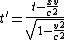(2{\it b})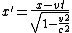(2{\it c})(2{\it d})#### Space-time geometry

A key question both in prerelativity physics and in special relativity is what quantities, describing the space-time relationships between events, are observer independent. Such quantities having observer-independent status may be viewed as describing the fundamental, intrinsic structure of space-time.

It has already been seen that in special relativity the time interval, Δt, between two events is no longer observer independent. Furthermore, since different inertial observers disagree over simultaneity, the spatial interval between two simultaneous events is not even a well-defined concept, and cannot be observer independent. Remarkably, however, in special relativity, all inertial observers agree upon the value of the space-time interval, I, between any two events, where I is defined by Eq. (3).

(3)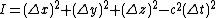What is most remarkable about this formula for I is that it is very closely analogous to the formula for squared distance in euclidean geometry. The minus sign occurring in the last term in Eq. (3) is of considerable importance, since it distinguishes between the notions of time and space in special relativity. Nevertheless, this minus sign turns out not to be a serious obstacle to the mathematical development of the theory of lorentzian geometry based upon the space-time interval, I, in a manner which parallels closely the development of euclidean geometry. In particular, notions such as geodesics (straightest possible lines) can be introduced in lorentzian geometry in complete analogy with euclidean geometry. The Lorentz transformation between the two inertial observers is seen from this perspective to be the mathematical analog of a rotation between two cartesian frames in euclidean geometry.

The formulation of special relativity as a theory of the lorentzian geometry of space-time is of great importance for the further development of the theory, since it makes possible the generalization which describes gravitation. The lorentzian geometry defined by Eq. (3) is a flat geometry, wherein initially parallel geodesics remain parallel forever. The theory of general relativity accounts for the effects of gravitation by allowing the lorentzian geometry of space-time to be curved. See Space-time

#### Consequences

The theory of special relativity makes many important predictions, the most striking of which concern properties of time. One such effect, known as time dilation, is predicted directly by the Lorentz transformation formula (2a). If observer carries a clock, then the event at which 's clock reads time &tgr; would be labeled by her as (&tgr;, 0, 0, 0). According to Eq. (2a), the observer would label the event as (t, x, 0, 0) where t is given by Eq. (4).

(4)Thus, could say that a clock carried by slows down on account of 's motion relative to . Similarly, would find that a clock carried by slows down with respect to hers. This apparent disagreement between and as to whose clock runs slower is resolved by noting that and use different notions is simultaneity in comparing the readings of their clocks.

The decay of unstable elementary particles provides an important direct application of the time dilation effect. If a particle is observed to have a decay lifetime T when it is at rest, special relativity predicts that its observed lifetime will increase according to Eq. (6) when it is moving. Exactly such an increase is routinely observed in experiments using particle accelerators, where particle velocities can be made to be extremely close to c. See Particle accelerator

An even more striking prediction of special relativity is the clock paradox: Two identical clocks which start together at an event A, undergo different motions, and then rejoin at event B will, in general, register different total elapsed time in going from A to B. This effect is the lorentzian geometry analog of the mundane fact in euclidean geometry that different paths between two points can have different total lengths. See Clock paradox

#### General theory

One of the basic tenets of special relativity is that no physical effect can propagate with a velocity greater than the speed of light, c, which represents a universal speed limit. On the other hand, classical gravitational theory describes the gravitational field of a body throughout space as a function of its instantaneous position, which is equivalent to the assumption that gravitational effects propagate with an infinite velocity. Thus, special relativity and classical gravitational theory are inconsistent, and a modified theory of gravity is necessary.

#### Principle of equivalence

It had long been considered a fundamental question why bodies of different mass fall with the same acceleration in a gravitational field. This situation was explained by Newton with the statement that both the gravitational force on a body and its inertial resistance to acceleration are proportional to its mass.

Newton's explanation is more in the nature of an ad hoc description. A deeper and more natural explanation occurred to Einstein. There are numerous forces other than gravity which are mass-proportional. These generally arise due to the use of accelerated coordinate systems to describe the motion, for example, the centrifugal force encountered in a rotating coordinate system. If an observer in the gravitational field of the Earth and another in an accelerating elevator or rocket in free space both drop a test body, they will both observe it to accelerate relative to the floor. According to classical theory, the Earth-based observer would attribute this to a gravitational force and the elevator-based observer would attribute it to the accelerated floor overtaking the uniformly moving body. In both cases the motion is identical, and in particular the acceleration is independent of the mass of the test body. Einstein elevated this fact to a general principle, the principle of equivalence; the principle states that on a local scale all physical effects of a gravitational field are indistinguishable from the physical effects of an accelerated coordinate system. This profound principle is the physical cornerstone of the theory of general relativity. From the point of view of the principle of equivalence, it is obvious why the motion of a test body in a gravitational field is independent of its mass. But the principle applies not only to mechanics but to all physical phenomena and thereby has profound consequences for electromagnetic and other nonmechanical phenomena. See Centrifugal force

#### Tensor field equations

The close connection between gravity and accelerating coordinate systems convinced Einstein that gravity is fundamentally a geometric phenomenon. Because of this, it is naturally described by the mathematics of higher-dimensional abstract geometry. This geometry involves systems of equations, called tensor equations, that are manifestly independent of the coordinate system. Tensors are a simple generalization of vectors.

The space-time of relativity contains one covariant second-rank tensor of particularly great importance, called the metric tensor gμ&ngr;, which is a generalization of the Lorentz metric of special relativity, introduced in Eq. (3). Nearby points in space-time, called events, which are separated by coordinate distances dxμ have an invariant physical separation whose square, called the line element, is defined by Eq. (5).

(5)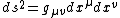This quantity is a generalization of the space-time interval, I, in special relativity.

Tensor equations are equations in which one tensor of a given type is set equal to another of the same type. The field equations of general relativity are tensor equations for the metric tensor, which completely describes the geometry of the space. The Riemann tensor (or curvature tensor), Rμβν α , plays a central role in the geometric structure of a space; if it is zero, the space is termed flat and has no gravitational field; if nonzero, the space is termed curved, and a gravitational field is present. In terms of the contracted Riemann tensor, that is, a Riemann tensor summed over α = β, the Einstein field equations for empty space are given by Eq. (6). (6)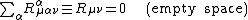The field equations are a set of 10 second-order partial differential equations since the four-by-four symmetric tensor Rμ&ngr; has 10 independent components; they are to be solved for the metric tensor. A solution in a given coordinate system defines an Einstein space-time. The curvature of this space corresponds to the intrinsic presence of a gravitational field. Thus the concept of a field of mechanical force in classical gravitational theory is replaced by the geometric concept of curved space in relativity theory.

In a nonempty region of space the field equations (6) must be modified to include a tensor representing the matter or energy content of space, the energy-momentum tensor Tμ&ngr;. The modified equations are Eq. (7), where

(7)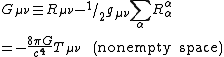G is the gravitational constant, equal to 6.67 × 10-11 N · m2 · kg-2. On the left is the tensor Gμ&ngr; representing the geometry of space, and on the right is the tensor Tμ&ngr; representing the mass or energy content of space. The tensor Gμ&ngr; defined in Eq. (7) is called the Einstein tensor. These equations automatically imply the conservation of energy and momentum, which is an extremely important result. Moreover, in the limiting case when the mass densities of all the gravitating bodies are small and their velocities are small compared to c, the equations reduce to the classical newtonian equations of gravity.

#### Cosmological term

The field equations were given in the form of Eq. (7) by Einstein in 1916. However, they can be consistently generalized by the addition of another term on the left side, which he called the cosmological term, &Lgr;gμ&ngr;. The more general equations are (8). The constant &Lgr; is called the cosmological constant.

(8)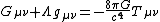Einstein introduced the cosmological term in 1917 in order to obtain mathematical models of the universe that were independent of time, since it was then believed that the universe was static. When it was discovered in 1929 that the universe is expanding, as evidenced by the Doppler shifts of distant galaxies, Einstein abandoned the cosmological term. However, interest in the cosmological constant has revived in connection with a serious inconsistency between relativity and quantum theory involving the quantum energy of the vacuum, and with observations since 1998 of type Ia supernovae which suggest that the expansion of the universe is accelerating.

#### Motion of test bodies

The path of a test body is a generalization of a straight line in euclidean space; it is the shortest “distance” (in terms of intervals ds) between points in space-time, known as a geodesic. General relativity theory possesses an extraordinary property: because the field equations are nonlinear, unlike those of newtonian theory, the motion of a test body in a gravitational field is not arbitrary since the body itself has mass and contributes to the field. Indeed, the field equations are so restrictive that the geodesic equation of motion is a necessary consequence and need not be treated as a separate postulate.

#### Schwarzschild solution

A very important solution of the field equations was obtained by K. Schwarzschild in 1916, surprisingly soon after the inception of general relativity. This solution represents the field in free space around a spherically symmetric body such as the Sun. It is the basis for a relativistic description of the solar system and most of the experimental tests of general relativity which have been carried out.

#### Gravitational redshift

Electromagnetic radiation of a given frequency emitted in a gravitational field will appear to an outside observer to have a lower frequency; that is, it will be redshifted. The redshift can be derived from the principle of equivalence. The most accurate test of the redshift to date was performed using a hydrogen maser on a rocket. Comparison of the maser frequency with Earth-based masers gave a measured redshift in agreement with theory to about 1 part in 104.

#### Perihelion shift

The equations of motion can be solved for a planet considered as a test body in the Schwarzschild field of the Sun. As should be expected, the orbits obtained are very similar to the ellipses of classical theory. However, the ellipse rotates very slowly in the plane of the orbit so that the perihelion, the point of closest approach of the planet to the Sun, is at a slightly different angular position for each orbit. This shift is extremely small. It is greatest in the case of the planet Mercury, whose perihelion advance is predicted to be 43 seconds of arc in a century. This agrees with the discrepancy between classical theory and observation, which was well known for many years before the discovery of general relativity.

#### Deflection of light

The principle of equivalence suggests an extraordinary phenomenon of gravity. Light or other electromagnetic radiation crossing the Einstein elevator horizontally will appear to be deflected downward in a parabolic arc because of the upward acceleration of the elevator. The same phenomenon must occur for light in the gravitational field of the Sun; it must be deflected toward the Sun. A calculation of this deflection gives 1.75 seconds for the net deflection of starlight grazing the edge of the Sun. Modern measurements, made by tracking quasars as they pass near or behind the Sun, find the deflection to be within 1% of the value predicted by general relativity.

In 1936 Einstein observed that if two stars were exactly lined up with the Earth, the more distant star would appear as a ring of light, distorted from its point appearance by the lens effect of the gravitational field of the nearer star. It was soon pointed out that a very similar phenomenon was much more likely to occur for entire galaxies instead of individual stars. Many candidates for such gravitational lens systems have been found.

In the curved space around the Sun the distance between points in space, for example between two planets, is not the same as it would be in flat space. In particular, the round-trip travel time of a radar signal sent between the Earth and the planet will be measurably increased by the curvature effect when the Earth, the Sun, and the planet are approximately lined up. Using a transponder on the Viking spacecraft, the time delay was found to agree with the predictions of general relativity to an accuracy of about one-half of 1%.

McGraw-Hill Concise Encyclopedia of Physics. © 2002 by The McGraw-Hill Companies, Inc.

## relativity

[‚rel·ə′tiv·əd·ē]
(physics)
Theory of physics which recognizes the universal character of the propagation speed of light and the consequent dependence of space, time, and other mechanical measurements on the motion of the observer performing the measurements; it has two main divisions, the special theory and the general theory.
McGraw-Hill Dictionary of Scientific & Technical Terms, 6E, Copyright © 2003 by The McGraw-Hill Companies, Inc.

## relativity

1. either of two theories developed by Albert Einstein, the special theory of relativity, which requires that the laws of physics shall be the same as seen by any two different observers in uniform relative motion, and the general theory of relativity which considers observers with relative acceleration and leads to a theory of gravitation
2. Philosophy dependence upon some variable factor such as the psychological, social, or environmental context
Collins Discovery Encyclopedia, 1st edition © HarperCollins Publishers 2005
References in periodicals archive ?
Science has marched inexorably onward in the 114 years since the publication of Special Relativity. And, since its the trademark of true science, Special Relativity has been constantly poked-at and challenged but has never been found wanting.
Einstein's theory of relativity nonetheless continues to survive tests and the most recent one involved the supermassive black hole at the center of the Milky Way.
Scientists already know from theoretical calculations that Chameleon Theory can reproduce the success of General Relativity in the solar system.
ING has gone live with Intelligent Voice and Relativity Trace in its Amsterdam location and is already monitoring almost 400 traders across email and IM, with voice following shortly.
Uploading images and production sets from ReadySuite directly into Relativity, including PDF and multi-page TIFF files which are auto-converted to Relativity's single-page TIFF requirement
"More than 100 years after he published his paper setting out the equations of general relativity, Einstein has been proved right once more - in a much more extreme laboratory than he could have possibly imagined," said the ESO in a statement.
But a recent discovery of a triple star system named PSR J0337+1715, located about 4,200 light-years from Earth provided a perfect laboratory scenario for an experiment to be conducted to test Einstein's theory of relativity in extreme gravity.
In order to facilitate the sale and address certain legacy liabilities of Relativity Media, the parties intend to implement the transaction though a section 363 sale under Chapter 11.
The long-term agreement with NASA grants Relativity exclusive use of the E4 test complex at Stennis to conduct engine testing around-the-clock, develop multiple vertical engine test stands, along with a second and first stage hold-down test stand.
Since the foundation of Relativity Gives, Relativity has provided more than USD 1.5 million dollars to Chicago Public Schools (CPS) through Wired to Learn and other giving initiatives to improve students' learning experiences and prepare them for college and careers through the use of educational technology.
The Relativity platform supports several languages and encodings for review, it is Unicode compliant and the analytics do work with things as complicated as traditional Chinese characters within the data hosted in Relativity.

Site: Follow: Share:
Open / Close